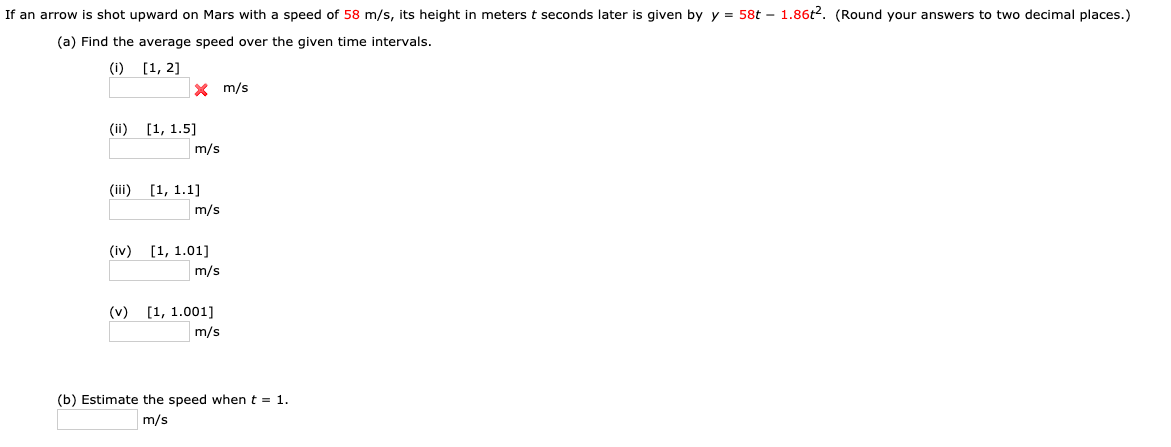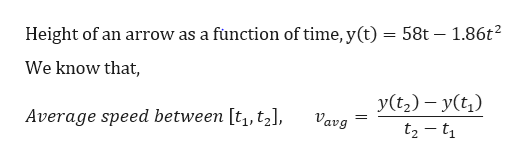# If an arrow is shot upward on Mars with a speed of 58 m/s, its height in meters t seconds later is given by y = 58t - 1.86t2. (Round your answers to two decimal places.)(a) Find the average speed over the given time intervals.(i) [1, 2]Xm/s(ii [1, 1.5m/s(iii) [1, 1.1m/s(iv)[1, 1.01]m/s(v) [1, 1.001]m/s(b) Estimate the speed when t = 1.m/s

Question
57 viewshelp_outlineImage TranscriptioncloseIf an arrow is shot upward on Mars with a speed of 58 m/s, its height in meters t seconds later is given by y = 58t - 1.86t2. (Round your answers to two decimal places.) (a) Find the average speed over the given time intervals. (i) [1, 2] Xm/s (ii [1, 1.5 m/s (iii) [1, 1.1 m/s (iv) [1, 1.01] m/s (v) [1, 1.001] m/s (b) Estimate the speed when t = 1. m/s fullscreen
check_circle

Step 1

NOTE: Hello there! As you have uploaded question with multiple sub-parts, as per our policy we will answer only the first three sub-parts for you. If you need help solving the other sub-parts, kindly re-upload them separately.

Step 2

Given,

...help_outlineImage TranscriptioncloseHeight of an arrow as a function of time, y(t) = 58t - 1.86t2 We know that y(t2) yt t2- ti Average speed between [t, t2], Vavg fullscreen

### Want to see the full answer?

See Solution

#### Want to see this answer and more?

Solutions are written by subject experts who are available 24/7. Questions are typically answered within 1 hour.*

See Solution
*Response times may vary by subject and question.
Tagged in
MathCalculus

### Other